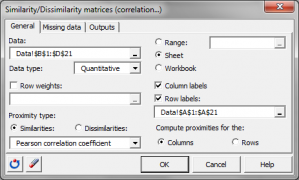# Similarity/Dissimilarity matrices (correlation…)

Computing similarity or dissimilarity among observations or variables can be very useful. Do it in Excel using the XLSTAT add-on statistical software.## What are Similarity and dissimilarity matrices

The proximity between two objects is measured by measuring at what point they are similar (similarity) or dissimilar (dissimilarity). The indexes offered depend on the nature of the data:

## Similarities and dissimilarities for quantitative data in XLSTAT

The similarity coefficients proposed by the calculations from the quantitative data are as follows: Cosine, Covariance (n-1), Covariance (n), Inertia, Gower coefficient, Kendall correlation coefficient, Pearson correlation coefficient, Spearman correlation coefficient.

The dissimilarity coefficients proposed by the calculations from the quantitative data are as follows:

• Bhattacharya's distance,
• Bray and Curtis' distance,
• Canberra's distance,
• Chebychev's distance,
• Chi² distance,
• Chi² metric,
• Chord distance,
• Squared chord distance,
• Euclidian distance,
• Geodesic distance,
• Kendall's dissimilarity,
• Mahalanobis distance,
• Manhattan distance,
• Ochiai's index,
• Pearson's dissimilarity,
• Spearman's dissimilarity.

## Similarities and dissimilarities for binary data in XLSTAT

The similarity and dissimilarity (per simple transformation) coefficients proposed by the calculations from the binary data are as follows:

• Dice coefficient (also known as the Sorensen coefficient),
• Jaccard coefficient,
• Kulczinski coefficient,
• Pearson Phi,
• Ochiai coefficient,
• Rogers & Tanimoto coefficient,
• Sokal & Michener's coefficient (simple matching coefficient),
• Sokal & Sneath's coefficient (1),
• Sokal & Sneath's coefficient (2).

## Similarities and dissimilarities for qualitative data in XLSTAT

The similarity coefficients proposed by the calculations from the qualitative data are as follows: Cooccurrence, Percent agreement.

The dissimilarity indexes proposed by the calculations from the qualitative data are as follows: Percent disagreement### analyze your data with xlstat

14-day free trial

Included in

Related features